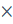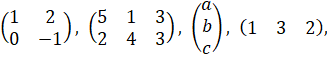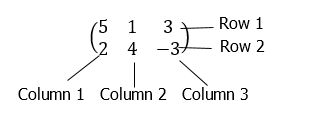### MATRICES

Mathematics S.S.S 3 First Term

WEEK 1

MATRICES I

Performance Objectives

Student should be able to:

1. Define matrix, identify matrix notation, identify different types of matrices.
2. Perform the operation of addition and subtraction of matrices.
3. Perform the scalar multiplication of 22 and 33 matrices.

Content

Matrix is a set of numbers arranged in rows and columns to form a rectangular array and enclosed within large brackets. These set of numbers enclosed within large brackets are called element or entries of the matrix.

The following are examples of matrices (plural of matrix)etc.

The matrixView More